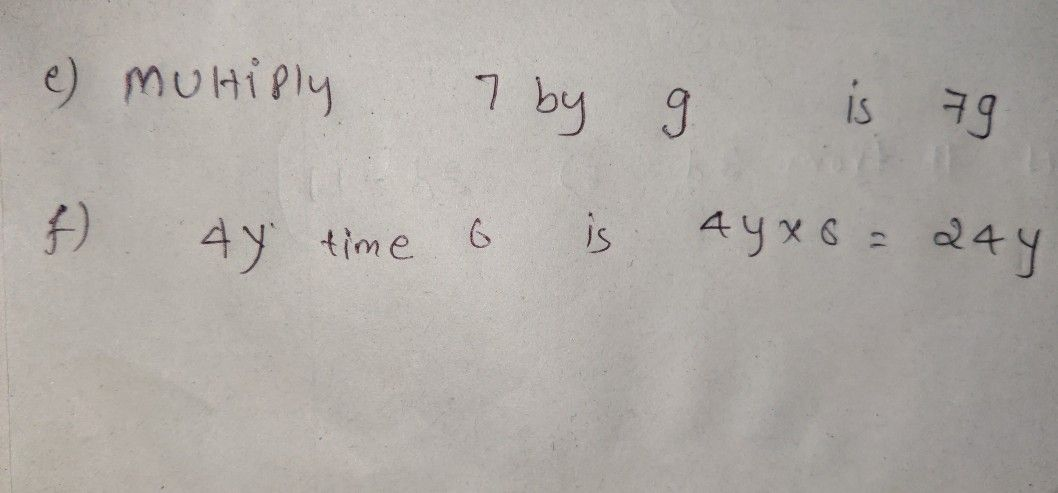Symbol
Probleme) $\right)$ Multiply $7$ $0y9$ $T\right)$ $4y$ times $6$